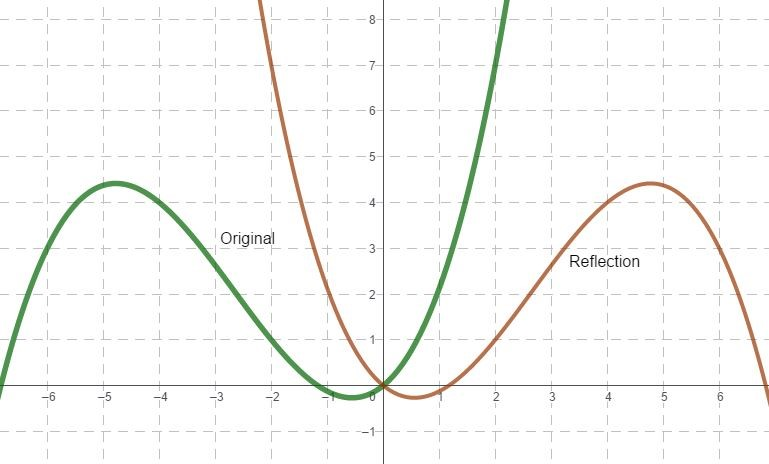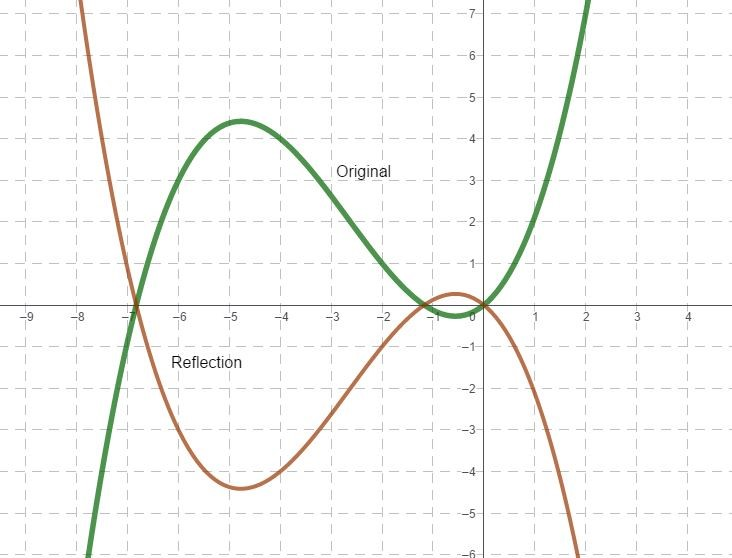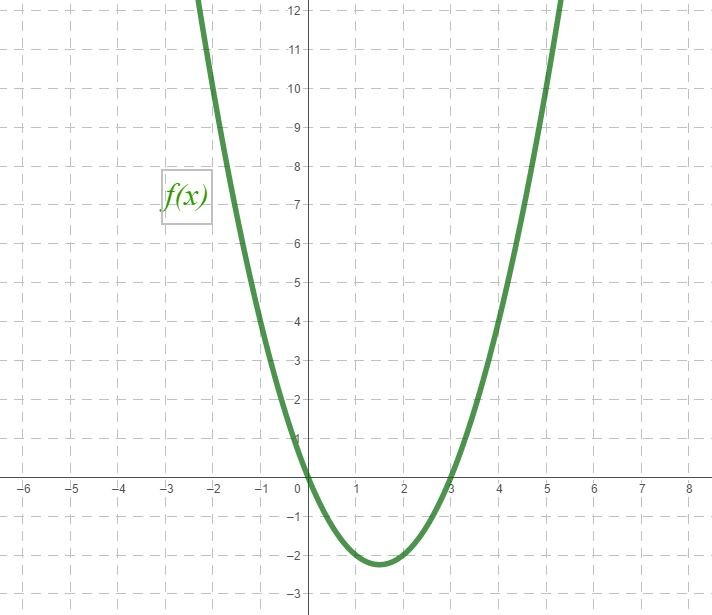# Transformation (Algebra Function)

In this section, we will study “how to transform a function, without evaluating its value”. After reading this article you will be able to understand transformation. You can translate, rotate or shift any graph easily.

## Contents

• Introduction
• Types of transformation
• Methodology
• Practical examples

## Introduction

Transformation is a basic technique to make graphs of function without evaluating their values. In mathematics whenever we want to check the behavior of any function, we have to draw its graph. Graph of any function is useful for checking its significance. Graphically representation of any function has much importance as its occurrence. Because without graphical representation, we cannot find out its importance.

Transformation of function helps us to study the functionality or feasibility of a function at a different stage. As we know that graphically representation of a function is important, then now we have to know how graphs are drawn?

To graph a function, first, we shall have to calculate different values of the function on different numbers, then sort out that data on the graph.

For example, if we want to draw the graph of function$f(x)=x+1$, then first we shall calculate some values of f(x) on different numbers for x. Then point out the pairs of values on the graph.

Let us calculate the function on different values of x.

For x = -2$f(1)=1-2=-1$

For x = -1$f(1)=1-1=0$

For x = 0$f(1)=1+0=1$

For x = 1$f(1)=1+1=2$

For x = 2$f(1)=1+2=3$

Now make the pairs in x,y form. Ordered pairs are given below,

(-2, -1), (-1, 0), (0, 1), (1, 2 ), (2, 3)

Now show these points on graph paper and sketch these points to make the graph.As seen in the figure, we first spot the ordered pair, then sketch the graph.

Now if we have to sketch the graph for$f(x)=2x+1$, then shall we start the whole procedure again? Yes! If we are not familiar with transformation of function or No! if we are familiar with the transformation of function.

So, now we can understand the importance of this topic. When we know how to transform a function, we do not have to do all these calculations.

One more thing, if you do not have a function just have a graph, then you can also convert this graph into the desired function.

As above graph for f(x) and we have to sketch graph for f(2x), then we just simply transform the graph by using key points of transformation.

There are many types of transformation, which we shall read in the next section.

## Types of transformations

There are three types of transformation for algebraic functions.

1. Translate
2. Reflect
3. Stretch

## Translate

In this type, the graph just shifts on the xy plane. Size and shape remain the same. In this type, we just shift the graph left or right on the x-axis or shift up and down on the y-axis.

There are two types of translation, one on the x-axis and the second is on the y-axis.

If$f(x)$ is the original graph, then$f(x+a)$ represents the shifted graph on the x-axis. If a is positive then the graph is shifted left by a unit on the x-axis and if a is negative then the graph is shifted right by a unit on the x-axis, As shown below.As in the above figure, the green graph is an original graph of f(x) and brown is translated graph of$f(x+4)$, which is shifted left by 4 units, similarly, the blue graph is translated graph of$f(x-4)$, which is shifted right by 4 units.

Secondly, if$f(x)$ is the original graph, then$f(x)+a$ represents the shifted graph on the y-axis. If a is positive then the graph shifted up by a units on the y-axis and if a is negative then the graph shifted down by a units on the y-axis, As shown below.As in the above figure, the green graph is the original graph of$f(x)$ and brown is translated graph of$f(x)+4$, which is shifted up by 4 units, similarly blue graph is translated graph of$f(x)-4$, which is shifted down by 4 units.

In both figures, we can see that the shape and size of the translated graph are the same, graphs are just shifted up, down, right, and left.

## Reflect

In this type of transformation, graphs are reflected across the axis. In this type, we just make the reflection of the graph according to the axis and the size of the graph remains the same.

There are also two types of reflections. One is along the x-axis and the second is along the y-axis.

If$f(x)$ is an original graph, then$f(-x)$ is a reflection along the y-axis. The graph will flip on the x-axis.

As shown in the figure.In the above figure, green is the original graph of$f(x)$ and brown is reflected graph of$f(-x)$. From the figure, the brown graph is a reflection of the green graph about the y-axis or flip on the x-axis.

Secondly, if$f(x)$ is an original graph, then$-f(x)$ is a reflection along the x-axis. The graph will flip on the y-axis.

As shown in the figure.In the above figure, green is the original graph of$f(x)$ and brown is reflected graph of$-f(x)$. From the figure, the brown graph is a reflection of the green graph about the x-axis or flip on the y-axis.

## Stretch

In this type of transformation, graphs change their look. The graph can be stretched in two ways, one is horizontally stretched, and the second is vertically stretched.

In horizontally starching y-axis remains the same. Horizontally starching of a graph for$f(x)$ is denoted by$f(ax)$. In this transformation, the y-axis remains the same, but x components are affected by a factor of$\frac{1}{a}$

As shown in the figure below.In the above figure, the green graph is the original graph of$f(x)$ and the brown graph is stretched graph of$f(2x)$. From the figure, it is cleared that the y-components of both graphs are the same, but components of x are affected by the factor of$\frac{1}{2}$

In vertically stretching x-axis remains the same. Vertically starching of a graph for$f(x)$ is denoted by$af(x)$. In this transformation, the x-axis remains the same, but y components are affected by a factor of a

As shown in the figure below.In the above figure, the green graph is the original graph of$f(x)$ and the brown graph is stretched graph of$2f(x)$. From the figure, it is cleared that the x-components of both graphs are the same, but components of y are affected by the factor of 2.

## Methodology

As we see above that there are three types of transformation. To make the transformation easy, we illustrate the transformation in two ways. One is x transformation and the second is y transformation.

x transformation

As we see in the previous examples, x-transformation behaves oppositely.

• Left Shifting,$f(x)\rightarrow f(x+a)$. This graph will shift on the left side of the x-axis by a units and y components remain unchanged.
• Right Shifting,$f(x)\rightarrow f(x-a)$. This graph will shift on the right side of the x-axis by a units and y components remain unchanged.
• Stretching along the x-axis or horizontal stretch,$f(x)\rightarrow f(ax)$. In this transformation graph stretch on the x-axis. x components are affected by the factor of$\frac{1}{a}$ and their components remain unchanged.
• Reflation in y-axis or flip on the x-axis,$f(x)\rightarrow f(-x)$. In this transformation graph flip along the x-axis. All the values of x components affected by the -ve sign and components of y remain unchanged.

y transformation

As we see in the above examples, y transformation takes place when we see change outside the brackets.

• Upward Shifting,$f(x)\rightarrow f(x)+a$. This graph will shift on the upside of the y-axis by a units and x components remain unchanged.
• Downward Shifting,$f(x)\rightarrow f(x)-a$. This graph will shift on the downside of the y-axis by a units and x components remain unchanged.
• Stretching along the y-axis or vertically stretch,$f(x)\rightarrow af(x)$. In this transformation graph stretch on the y-axis. y components are affected by the factor of a and the x components remain unchanged.
• Reflation in x-axis or flip on the y-axis,$f(x)\rightarrow -f(x)$. In this transformation graph flip along the y-axis. All the values of y components affected by the -ve sign and components of x remain unchanged.

Always remember, changes in brackets lead us to transformation on the x-axis, and changed outside the brackets mean transformation on the y-axis.

## Practical Examples

Problem 1: Graph of$f(x)=x^{2}-3x$, is given below. Without evaluating the value plot the graph of$f(2x)+1$ on the same axis.Since we have to transform the$f(x)$ into$f(2x)+1$. Here we have to transform both the x and y-axis. Inside the bracket means transformation on the x-axis and outside the bracket means transformation on the y-axis.

First, start from x transformation.

According to the required function, we have to stretch the graph horizontally by the factor of$\frac{1}{2}$, because required function is$f(2x)$. Values of y components remain unchanged and values of x component affected by the factor of$\frac{1}{2}$

As shown below.In the figure, it is clear that$f(x)$ is cutting the x-axis at x = 3, but after the transformation of$f(2x)$ graph cutting the x-axis at x = 1.5, because x components are affected by the factor of$\frac{1}{2}$.

Now we transform the y-axis. For$f(2x)+1$ we shift the graph upward on the y-axis, components of y will be affected by 1 unit, and components of x will remain unchanged.

As shown below.Brown graph shifted upward by 1 unit, represented by the blue graph.

So blue graph is our required graph of$f(2x)+1$.

Source:

AS Level Mathematics Textbook by Aaran Karia
Pure mathematics 1 by Sophie Goldie